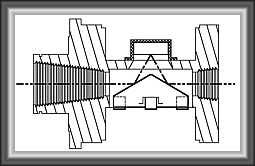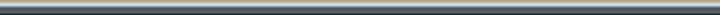CalculatorsPrevious page | Home

Engineering Units Conversion Calculator
Select the units that you have, input the number, then select the units you want to convert to.

Convert From:

Number:

Convert to:

*

Convert From:

Number:

Convert to:

*

Convert From:

Number:

Convert to:

*

Convert From:

Number:

Convert to:

*

Convert From:

Number:

Convert to:

*

Convert From:

Number:

Convert to:

*

Convert From:

Number:

Convert to:

*

Convert From:

Number:

Convert to:

*

Convert From:

Number:

Convert to:

*

Coefficient of Heat Transfer Conversion Factors

Convert From:

Number:

Convert to:

*Previous page | Home